Example: Evaluate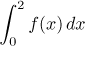, where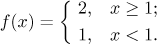Solution: This function looks like this: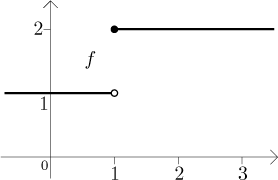We have to decide on partitions. Note that in rectangles that do not involve the point x = 1, the function f is constant. This means that the supremum and infimum are equal there, in other words, the upper and lower sums agree at those parts. The only difference between the upper and lower sum will happen around x = 1, so we will chose partitions that ignore the constant parts and focus on x = 1: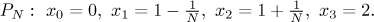We look at the pictureand see that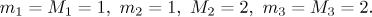Thus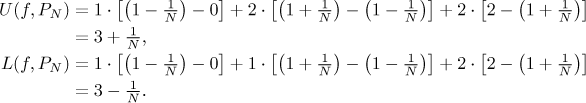The upper and lower sum can be also directly seen from the picture, the shaded area on the left is the upper sum, on the right the lower sum: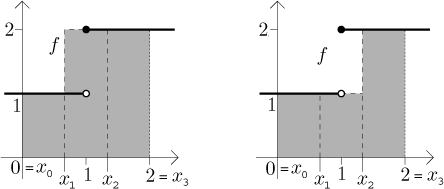We therefore get bounds for infimum and supremum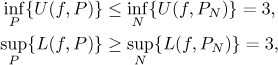and so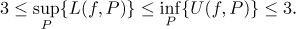Consequently,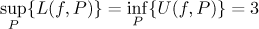and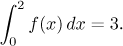This answer seems clear from the picture, the region consists of three squares of side one.